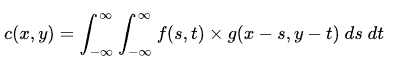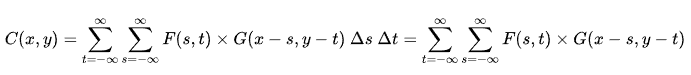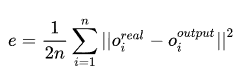• GNN:图神经网络用于粒子探测器事件数据中的噪声分类Python
• 线性神经网络 x=-5:5; y=3*x-7; randn('state',2); % 设置种子，便于重复执行 y=y+randn(1,length(y))*1.5; % 加入噪声的直线 plot(x,y,'o'); P=x;T=y; lr=maxlinlr(P,'bias') % 计算最大学习率 加入b偏置 ...
线性神经网络
x=-5:5;
y=3*x-7;
randn('state',2);			% 设置种子，便于重复执行
y=y+randn(1,length(y))*1.5;		% 加入噪声的直线
plot(x,y,'o');
P=x;T=y;
lr=maxlinlr(P,'bias')			% 计算最大学习率 加入b偏置
net=linearlayer(0,lr);			% 用linearlayer创建线性层，输入延迟为0
tic;net=train(net,P,T);toc		% 用train函数训练
new_x=-5:.2:5;
new_y=sim(net,new_x);           	% 仿真
hold on;plot(new_x,new_y);
title('linearlayer用于最小二乘拟合直线');
legend('原始数据点','最小二乘拟合直线');
xlabel('x');ylabel('y');
s=sprintf('y=%f * x + %f', net.iw{1,1}, net.b{1,1})  %w值第一个为偏置的权值(net.b)，第二个为x.x的权值(net.iw{1,1})第三个为x.y的权值
text(-2,0,s);

非线性神经网络  添加非线性成分后的线性神经网络用于求解异或逻辑
% xor_linearlayer.m
%% 清理
close all
clear,clc
%% 定义变量
P1=[0,0,1,1;0,1,0,1]            	% 原始输入向量
p2=P1(1,:).^2;
p3=P1(1,:).*P1(2,:);
p4=P1(2,:).^2;
P=[P1(1,:);p2;p3;p4;P1(2,:)]    	% 添加非线性成分后的输入向量
d=[0,1,1,0]                     	% 期望输出向量
lr=maxlinlr(P,'bias')			% 根据输入矩阵求解最大学习率
%% 线性网络实现
net=linearlayer(0,lr);          	% 创建线性网络
net=train(net,P,d);             	% 线性网络训练
%% 显示
disp('网络输出')                	% 命令行输出
Y1=sim(net,P)
disp('网络二值输出');
YY1=Y1>=0.5
disp('最终权值：')
w1=[net.iw{1,1}, net.b{1,1}]
plot([0,1],[0,1],'o','LineWidth',2);    % 图形窗口输出
hold on;
plot([0,1],[1,0],'d','LineWidth',2);
axis([-0.1,1.1,-0.1,1.1]);
xlabel('x');ylabel('y');
title('线性神经网络用于求解异或逻辑');
x=-0.1:.1:1.1;y=-0.1:.1:1.1;
N=length(x);
X=repmat(x,1,N);  %repmat(A,m,n)功能是以A的内容堆叠在（MxN）
Y=repmat(y,N,1);Y=Y(:);Y=Y';
P=[X;X.^2;X.*Y;Y.^2;Y];
yy=net(P);
y1=reshape(yy,N,N);
[C,h]=contour(x,y,y1,0.5,'b');
clabel(C,h);
legend('0','1','线性神经网络分类面');

% xor_madaline.m  Madaline用于求解异或逻辑吧 损失函数物理意义划分错误的点到超平面的的距离和
%% 清理
close all
clear,clc
%% 第一个神经元
P1=[0,0,1,1;0,1,0,1];               % 输入向量
d1=[1,0,1,1];                       % 期望输出向量
lr=maxlinlr(P1,'bias');             % 根据输入矩阵求解最大学习率
net1=linearlayer(0,lr);             % 创建线性网络
net1=train(net1,P1,d1);             % 线性网络训练
%% 第二个神经元
P2=[0,0,1,1;0,1,0,1];               % 输入向量
d2=[1,1,0,1];                       % 期望输出向量
lr=maxlinlr(P2,'bias');             % 根据输入矩阵求解最大学习率
net2=linearlayer(0,lr);             % 创建线性网络
net2=train(net2,P2,d2);             % 线性网络训练
Y1=sim(net1,P1);Y1=Y1>=0.5;
Y2=sim(net2,P2);Y2=Y2>=0.5;
Y=~(Y1&Y2);
%% 显示
disp('第一个神经元最终权值：')      % 命令行输出
w1=[net1.iw{1,1}, net1.b{1,1}]
disp('第二个神经元最终权值：')
w2=[net2.iw{1,1}, net2.b{1,1}]
disp('第一个神经元测试输出：')
Y1
disp('第二个神经元测试输出：');
Y2
disp('最终输出：');
Y
plot([0,1],[0,1],'bo');             % 图形窗口输出
hold on;
plot([0,1],[1,0],'d');
x=-2:.2:2;
y1=1/2/w1(2)-w1(1)/w1(2)*x-w1(3)/w1(2);   % 第一条直线,1/2是区分0和1的阈值  %net1.iw{1,1}(1)*x+net1.iw{1,1}(2)*y+net1.b{1,1}=1/2(阈值)
plot(x,y1,'-');
y2=1/2/w2(2)-w2(1)/w2(2)*x-w2(3)/w2(2);   % 第二条直线，1/2是区分0和1的阈值
plot(x,y2,'--');
axis([-0.1,1.1,-0.1,1.1])
xlabel('x');ylabel('y');
title('Madaline用于求解异或逻辑')
legend('0','1','第一条直线','第二条直线');
展开全文• 对两种神经网络在训练时间、识别精度和受噪声影响三方面作以比较,发现概率神经网络在训练时间方面有优势,而自组织竞争神经网络在有噪声情况下识别精度较高,故建议在大型结构健康监测系统中确定损伤位置时将二者联合...
• 针对脉冲耦合神经网络（PCNN）的降噪效果差和参数不确定性的问题，提出了一种采用灰狼优化（GWO）和二维经验模态分解（BEMD）优化的自适应PCNN的混合图像去噪方法。 ）， 被表达。 BEMD用于将原始图像分解为多层图像...
• 用于回归的神经网络写法（基于make_wave数据集）前言数据展示以及准备经典算法第一种神经网络写法第二种神经网络写法第三种神经网络写法结论 前言 本文本适合于学习神经网络的小白，高手或大神请绕行。 上一集我做了...
用于回归的神经网络写法（基于make_wave数据集）前言数据展示以及准备经典算法第一种神经网络写法第二种神经网络写法第三种神经网络写法结论
前言
本文本适合于学习神经网络的小白，高手或大神请绕行。
上一集我做了使用iris（鸢尾花分类）数据集进行一个神经网络分类的学习，这次使用一个虚拟产生的数据集（make_wake）进行神经网络回归的学习。
make_wake数据集是使用mglearn库产生一系列叠加在线性数据上带噪声波形分布数据。
大概意思如下图。因此，我们通过上述输入的x特征来预测输出的y，即进行回归模拟。
数据展示以及准备
环境：python3.7+keras2.3.1+tensorflow1.15.0+sklearn0.22.1+matplotlib3.1.1
三方库：mglearn0.1.7
为什么使用mglearn呢？因为它已经封装好了matplotlib.pyplot的scatter方法，只输入X， y就好了，学习时真的没有必要重复造轮子。
本博客程序代码wave_data_training.ipynb

首先导入通用库

# import common
from sklearn.model_selection import train_test_split
from sklearn.metrics import r2_score
from mglearn.datasets import make_wave
import numpy as np
import matplotlib.pyplot as plt
import mglearn


加载数据

# get data
X, y = make_wave(n_samples=150)
print('Shape', X.shape, y.shape)
RANDOM_STATE = 2


查看数据分布

mglearn.discrete_scatter(X, y)在这里，我们写一个展示真实结果与预测结果的比对的方法。即分别是看一下数据区间内回归结果曲线，对比训练集真实点和预测点的位置，对比测试集真实点和预测点的位置。
def show_plot(X_pred_line, y_pred_line, X_train, y_train, y_train_pred, X_test, y_test, y_test_pred):
f = plt.figure()
plt.title('True and Predict')
mglearn.discrete_scatter(X, y, labels=['True Point'], s=5)
lines = plt.plot(X_pred_line, y_pred_line, c='red', label='Predict Line')
plt.legend()
f = plt.figure()
plt.title('Train set')
mglearn.discrete_scatter(X_train, y_train, s=5, c='blue', labels=['True Train Point'])
mglearn.discrete_scatter(X_train, y_train_pred, s=5, c='red', labels=['Predict Train Point'])
plt.legend()
f = plt.figure()
plt.title('Test set')
mglearn.discrete_scatter(X_test, y_test, s=5, c='blue', labels=['True Test Point'])
mglearn.discrete_scatter(X_test, y_test_pred, s=5, c='red', labels=['Predict Test Point'])
plt.legend()

经典算法
学习机器学习的小伙伴一定知道，使用线性回归模型几乎是入门第一课。但今天我们不使用这个，反正在sklearn里，不外乎就是创建对象，fit数据，预测结果，所以我们看看支持向量机（Support Vector Machine， SVM）的拟合结果。SVM的其实最原本是使用于分类问题，而且还是二分类问题，SVC（分类）算法在两种类别之间找出一个超平面，使两种类别数据集分别离这个平面最远，离这个超平面最近的点就是支持向量。而SVR（回归）算法是找出一个超平面，使数据集上的点到这个平面的距离最近。具体公式和原理这里就不详细展开了，毕竟不是给大佬们看的。
# import common
from sklearn.svm import SVR
X_train, X_test, y_train, y_test = train_test_split(X, y, random_state=RANDOM_STATE)
svr = SVR(C=10, gamma='auto')
svr.fit(X_train, y_train)
# svr.score(X_train, y_train)
# svr.score(X_test, y_test)
y_train_pred = svr.predict(X_train)
y_test_pred = svr.predict(X_test)
print('Train score:', r2_score(y_train, y_train_pred))
print('Test score:', r2_score(y_test, y_test_pred))

X_pred_line = np.linspace(X.min(), X.max(), 100)
y_pred_line = svr.predict(X_pred_line.reshape(-1, 1))

show_plot(X_pred_line, y_pred_line, X_train, y_train, y_train_pred, X_test, y_test, y_test_pred)

sklearn里使用SVR十分方便，只要用SVR创建一个对象，fit一下数据，就可以做预测了。
这里的结果是
Train score: 0.7673734917507331
Test score: 0.7901620865051271毕竟数据量小，结果可以大概看出分布趋势。
第一种神经网络写法
sklearn的使用方便让人无法抗拒，只是创建一下，fit一下，就可以预测了（当然有要调超参的，以后再写GridSearchCV）。
而sklearn里有神经网络的模型，可以直接调用。
# import common
from sklearn.neural_network import MLPRegressor

MLPClassifier是多层感知机，即我们所说的神经网络，不过它只都是全连接层。实现方法，同样是创建，fit，预测即可。
X_train, X_test, y_train, y_test = train_test_split(X, y, random_state=RANDOM_STATE)
mlp = MLPRegressor(hidden_layer_sizes=(50, 50, 50, 50, 50, 50, 50, 50), activation='relu', max_iter=100000, verbose=0, tol=0.0001)  # tol=0.00001 overfitting, hidden_layer_sizes=50 easy to underfitting
mlp.fit(X_train, y_train)

# mlp.score(X_train, y_train)
# mlp.score(X_test, y_test)
y_train_pred = mlp.predict(X_train)
y_test_pred = mlp.predict(X_test)
print('Train score:', r2_score(y_train, y_train_pred))  # best 0.78
print('Test score:', r2_score(y_test, y_test_pred))  # best 0.76

X_pred_line = np.linspace(X.min(), X.max(), 100)
y_pred_line = mlp.predict(X_pred_line.reshape(-1, 1))

show_plot(X_pred_line, y_pred_line, X_train, y_train, y_train_pred, X_test, y_test, y_test_pred)

这里的结果：
Train score: 0.819730694026285
Test score: 0.6920529004328322可以看到，使用这个方法有点过拟合，有可能是我使用了比较多的隐藏层，但使用很简单。
第二种神经网络写法
刚才第一种神经网络写法还是比较简单，这次可以自主搭建一下神经网络——使用Keras。
Keras是将下层深度神经网络封装成高级应用API，然后使用最简单的办法去调用，减少编程的难度
# import common
import keras
from keras.layers import Dense, Activation, Dropout
from keras.losses import mean_squared_error
from keras.models import Sequential
from keras.optimizers import Adam, SGD

首先，我们的输入和输出都是1维数据，十分简单。然后使用其中一种方式搭配网络：（输入层1维，有5个隐藏网络层，都有50个单元，都经过一个激活函数tanh，同时也使用Dropout（即随机忽略一个单），最后就是输出层1维，最后使用tanh激活函数）。
注：最后的输出一个值即可。
神经网络的训练方法：
网络模型->优化方法->损失函数->数据喂养->预测结果
X_train, X_test, y_train, y_test = train_test_split(X, y, random_state=RANDOM_STATE)

model = Sequential()
model.add(Dense(50, input_shape=(X_train.shape, )))
model.add(Activation('tanh'))
model.add(Dropout(0.1))  # less and drop less, use Dropout the result uncencern
model.add(Dense(50))
model.add(Activation('tanh'))
model.add(Dropout(0.1))  # less and drop less, use Dropout the result uncencern
model.add(Dense(50))
model.add(Activation('tanh'))
model.add(Dropout(0.1))  # less and drop less, use Dropout the result uncencern
model.add(Dense(50))
model.add(Activation('tanh'))
model.add(Dropout(0.1))  # less and drop less, use Dropout the result uncencern
model.add(Dense(50))
model.add(Activation('tanh'))
model.add(Dense(1))
model.add(Activation('tanh'))
model.compile(Adam(), mean_squared_error, ['mse'])
# model.compile(SGD(learning_rate=0.01, momentum=0.9, nesterov=True), mean_squared_error, ['mse'])
history = model.fit(X_train, y_train, batch_size=200, epochs=1000, verbose=0)
f = plt.figure()
plt.title('Loss')
plt.plot(history.history['loss'])

y_train_pred = model.predict(X_train)
y_test_pred = model.predict(X_test)
print('Train score:', r2_score(y_train, y_train_pred))  # best 0.74
print('Test score:', r2_score(y_test, y_test_pred))  # best 0.77

X_pred_line = np.linspace(X.min(), X.max(), 100)
y_pred_line = model.predict(X_pred_line.reshape(-1, 1))

show_plot(X_pred_line, y_pred_line, X_train, y_train, y_train_pred, X_test, y_test, y_test_pred)

最终结果：
Train score: 0.6915371066622927
Test score: 0.678329816849878使用自己搭建网络，结果是随机的，有时比较好，有时比较差。
较好时的结果：
Train score: 0.7254240148497262
Test score: 0.7132507669617197这里的Keras搭建网络多种方式，请参考以前写的Keras添加网络层的N种方法。
第三种神经网络写法
这一种方法是目前最复杂的（当然，你可以的话可以去写深度学习的框架，再用你的框架来搭建神经网络），使用tensorflow搭建神经网络。
这里的神经网络的训练方法：
输入输出占位符->网络模型（前向传播的方法）->损失函数->优化方法优化损失值->数据喂养->预测结果
# import common
import tensorflow as tf
X_train, X_test, y_train, y_test = train_test_split(X, y, random_state=RANDOM_STATE)
y_train_c = y_train.reshape(-1, 1)
y_test_c = y_test.reshape(-1, 1)

INPUT_NODE = 1
LAYER1 = 50
LAYER2 = 50
LAYER3 = 50
LAYER4 = 50
LAYER5 = 50
OUTPUT_NODE = 1
tf.reset_default_graph()
def feed_forward(input_tensor):
weight1 = tf.get_variable('weight1', [INPUT_NODE, LAYER1], initializer=tf.truncated_normal_initializer(stddev=0.1))
bias1 = tf.get_variable('bias1', [LAYER1], initializer=tf.constant_initializer(0.0))
weight2 = tf.get_variable('weight2', [LAYER1, LAYER2], initializer=tf.truncated_normal_initializer(stddev=0.1))
bias2 = tf.get_variable('bias2', [LAYER2], initializer=tf.constant_initializer(0.0))
weight3 = tf.get_variable('weight3', [LAYER2, LAYER3], initializer=tf.truncated_normal_initializer(stddev=0.1))
bias3 = tf.get_variable('bias3', [LAYER3], initializer=tf.constant_initializer(0.0))
weight4 = tf.get_variable('weight4', [LAYER3, LAYER4], initializer=tf.truncated_normal_initializer(stddev=0.1))
bias4 = tf.get_variable('bias4', [LAYER4], initializer=tf.constant_initializer(0.0))
weight5 = tf.get_variable('weight5', [LAYER4, LAYER5], initializer=tf.truncated_normal_initializer(stddev=0.1))
bias5 = tf.get_variable('bias5', [LAYER5], initializer=tf.constant_initializer(0.0))
weightout = tf.get_variable('weightout', [LAYER5, OUTPUT_NODE], initializer=tf.truncated_normal_initializer(stddev=0.1))
biasout = tf.get_variable('biasout', [OUTPUT_NODE], initializer=tf.constant_initializer(0.0))
layer1 = tf.nn.relu(tf.matmul(input_tensor, weight1) + bias1)
layer2 = tf.nn.relu(tf.matmul(layer1, weight2) + bias2)
layer3 = tf.nn.relu(tf.matmul(layer2, weight3) + bias3)
layer4 = tf.nn.relu(tf.matmul(layer3, weight4) + bias4)
layer5 = tf.nn.relu(tf.matmul(layer4, weight5) + bias5)
layerout = tf.matmul(layer5, weightout) + biasout
return layerout

LEARNING_RATE = 0.01
TRAINING_TIMES = 5000
def train():
X_input = tf.placeholder(tf.float32, [None, INPUT_NODE], 'X_input')
y_output = tf.placeholder(tf.float32, [None, OUTPUT_NODE], 'y_output')
y_inference = feed_forward(X_input)
print('Shape', y_output.shape, y_inference.shape)

loss = tf.reduce_mean(tf.square(y_output - y_inference))
train_op = tf.train.AdamOptimizer(LEARNING_RATE).minimize(loss)

X_pred_line = np.linspace(X.min(), X.max(), 100)

with tf.Session() as sess:
tf.global_variables_initializer().run()
for i in range(TRAINING_TIMES):
_, lossend = sess.run([train_op, loss], feed_dict={X_input: X_train, y_output: y_train_c})
if i % 1000 == 0:
print('After train {} times, loss is {}'.format(i, lossend))
y_val = sess.run(y_inference, feed_dict={X_input: X_test})
print('Score:', r2_score(y_test_c, y_val))

y_pred_line = sess.run(y_inference, feed_dict={X_input: X_pred_line.reshape(-1, 1)})

y_train_pred = sess.run(y_inference, feed_dict={X_input: X_train})
y_test_pred = sess.run(y_inference, feed_dict={X_input: X_test})
print('Train score:', r2_score(y_train, y_train_pred))
print('Test score:', r2_score(y_test, y_test_pred))

train()
show_plot(X_pred_line, y_pred_line, X_train, y_train, y_train_pred, X_test, y_test, y_test_pred)

在这里提一下，使用tf.reset_default_graph()的原因，如果是使用文件每次直接运行，不存在问题。如果在jupyter notebook里运行，在第二次后会报错，提醒你tensor的变量不可用因为已经定义使用过了。出现这个问题需要你restart jupyter notebook，所以重置计算图就不需要restart jupyter notebook了。
结果如下：
After train 0 times, loss is 1.1412855386734009
Score: 0.03146116885653394
After train 1000 times, loss is 0.17113439738750458
Score: 0.5452508638546051
After train 2000 times, loss is 0.11893297731876373
Score: 0.5308275700994873
After train 3000 times, loss is 0.08823881298303604
Score: 0.371095879467622
After train 4000 times, loss is 0.0871317982673645
Score: 0.2086708416853813
Train score: 0.933845165631305
Test score: 0.27019577421318也是可以得到相同的结果。结果是随机的，有时比较好，有时比较差。
结论
本文使用三个不同的方法使用神经网络，那么对于简单的问题，当然可以使用简单的方法，但遇到复杂的问题，还是老实地使用Keras或tensorflow吧。


展开全文深度学习 机器学习
• 卷积神经网络可以分为离散卷积神经网络和连续卷积神经网络。 卷积是计算机视觉、图像处理、数字信号、人工智能等很多领域里常用的算法。比如，它可以在图像处理中用于钝化一幅图片，这时我们运用的是二维均值离散...
卷积神经网络可以分为离散卷积神经网络和连续卷积神经网络。

卷积是计算机视觉、图像处理、数字信号、人工智能等很多领域里常用的算法。比如，它可以在图像处理中用于钝化一幅图片，这时我们运用的是二维均值离散卷积。。卷积减弱了原来图片中的噪声，但也降低了图片的清晰度。

连续卷积公式：简单地说明：

c(x,y)：神经网络输出矩阵；

f(s,t)：权值矩阵，在一些书上叫做滤波器，或者掩膜；不同的滤波器不同的作用；

g(x-s,y-t)：输入矩阵；

这式子的含义是：遍览从负无穷到正无穷的全部 s 和 t 值，把 g 在 (x-s, y-t) 上的值乘以 f 在 (s, t) 上的值之后再“加和”到一起（积分意义上），得到 c 在 (x, y) 上的值。

离散卷积神经网络：公式的意思是，以（x，y）为中心，对输入矩阵距离中心（-s，-y）的值进行加权计算，然后将计算得到的值放在（x，y）的位置上，以此类推，计算下一个数。在这里我们把dertx,derty看作1。

卷积的过程大致如下：把权值矩阵的中心元素对准输入矩阵的第一个元素，把权值矩阵与输入矩阵重合的对应元素相乘相加，再把结果写入输出矩阵的第一个元素；然后把权值矩阵移动到输入元素的第二个元素，同样把所有重合元素相乘相加后写入对应的输出矩阵的第二个元素；重复这样的操作直到权值矩阵移过了所有的输入矩阵元素。权值矩阵的值事实上是每一次求和时输入元素的权，即矩阵中每个元素的周围元素对它影响的程度，加权求和即将这种影响施加到了正在计算的元素。可以想见，如果权值矩阵只有中心元素为1，其他元素都是零，则卷积之后输出矩阵与输入矩阵是等同的。

注意：

1.F 是上下左右翻转后再与 G 对准的。因为卷积公式中 F(s, t) 乘上的是 G(x-s, y-t) 。比如 F(-1, -1) 乘上的是 G(7, 7) ；

2,.如果 F 的所有值之和不等于 1.0，则 C 值有可能不落在 [0, 255] 区间内，那就不是一个合法的图像灰度值。所以如果需要让结果是一幅图像，就得将 F 归一化——令它的所有位置之和等于 1.0 ；

3.对于 G 边缘上的点，有可能它的周围位置超出了图像边缘。此时可以把图像边缘之外的值当做 0 。或者只计算其周围都不超边缘的点的 C 。这样计算出来的图像就比原图像小一些。在上例中是小了一圈，如果 F 覆盖范围更大，那么小的圈数更多。

不同的滤波器有不同的作用，如果随机产生一个权值矩阵，那么会有不同的效果，但是，不能对应每一个功能设计一个滤波器，这样的工作量就有点大了，所以，人们不经会想，如果不是由人来设计一个滤波器，而是从一个随机滤波器开始，根据某种目标、用某种方法去逐渐调整它，直到它接近我们想要的样子，可行么？这就是卷积神经网络（Convolutional Neural Network, CNN）的思想了。可调整的滤波器是 CNN 的“卷积”那部分；如何调整滤波器则是 CNN 的“神经网络”那部分。

要达到这个目的，首先准备一个从目标函数采样的包含若干“输入－输出对”的集合——训练集。把训练集的输入送给神经网络，得到的输出肯定不是正确的输出。因为一开始这个神经网络的行为是随机的。

把一个训练样本输入给神经网络，计算输出与目标输出的（向量）差的模平方（自己与自己的内积）。再把全部 n 个样本的差的模平方求平均，得到 e ：e 称为均方误差 mse 。全部输出向量和目标输出向量之间的距离（差的模）越小，则 e 越小。e 越小则神经网络的行为与想要的行为越接近。我们目标是使 e 变小。在这里 e 可以看做是全体权值和偏置值的一个函数。这就成为了一个无约束优化问题。如果能找到一个全局最小点，e 值在可接受的范围内，就可以认为这个神经网络训练好了。它能够很好地拟合目标函数。这里待优化的函数也可以是 mse 外的其他函数，统称 Cost Function，都可以用 e 表示。

在 卷积神经网络 中，这样的滤波器层叫做卷积层。一个卷积层可以有多个滤波器，每一个叫做一个 channel 。图像是二维信号。信号也可以是其他维度的，比如一维、三维乃至更高维度。那么滤波器相应的也有各种维度。回到二维图像的例子，实际上一个卷积层面对的是多个 channel 的 “一摞” 二维图像。
展开全文• 论文链接：https://openreview.net/pdf?id=B1oK8aoxe1. 引言近期，深度强化学习取得了许多令人印象深刻的结果，但这些深度强化学习算法一般都是采用单纯探索策略，如 ε-贪婪算法或统一的高斯探索噪声，这些方法在...论文链接：https://openreview.net/pdf?id=B1oK8aoxe1. 引言近期，深度强化学习取得了许多令人印象深刻的结果，但这些深度强化学习算法一般都是采用单纯探索策略，如 ε-贪婪算法或统一的高斯探索噪声，这些方法在奖励稀疏的任务中表现不佳。要想解决这些问题，可采用两种策略：1. 设计动作的层次结构，这需要专业知识以及细致的手动工程。2. 使用与领域无关的内在奖励来指导探索，然而，对于如何将一个任务的知识进行转化以解决其他任务尚不清楚，这可能会导致过高的样本复杂性。本文作者提出了一个可以在预训练环境中学习一系列技能的通用框架，该框架可以通过在这些技能上训练高级策略再应用于下游项目。作者利用随机神经网络（SNN）与代理奖励（proxy reward）相结合来学习这些技能，这样的设计不需要太多与下游项目相关的知识。为了鼓励 SNN 策略的行为多样性，研究者在预训练阶段提出了一种基于互信息（MI）的信息理论正则器。2. 问题描述本论文作者通过一组时域有限的离散时间折扣马尔可夫决策过程（MDPs）M 指定了一组下游任务。目标是在整个过程中实现期望折扣回报的最大化。为了解决这些问题，首先这些下游项目要满足一些结构性假设，这是确保泛化性能的最低要求。正如智能体空间的思想，状态空间可以分为两部分：智能体状态和休息状态，这两个状态间的相互作用是很弱的。在 M 中，智能体状态对 M 中的 MDP 都是一样的，所有的 MDP 都要共享相同的动作空间。建立了一系列满足结构假设的任务后，目标是要最小化解决这些任务所需的全部样本的复杂性。之前的技术是利用解决前期任务收集到经验来帮助解决后面的任务，但这并不适用于奖励稀疏的任务。因而研究者提出了一个在预训练环境中学习有用技能的通用框架。3.方法论作者用 5 步过程描述了该方法。该方法利用了预训练任务的优势，而且是用最少的专业知识来构建，同时可以学得很多有用的技能来解决稀疏奖励的极具挑战的任务。3.1 建立预训练环境为了建立智能体学习可用于下游任务的有用技能的预训练环境，作者允许智能体在最小设置下自由地与环境进行交互。例如，对移动机器人来说，预训练环境会是一个宽敞的环境，机器人可以先在这个环境中学到必要的移动能力。与在预训练环境中指定特定技能的目标不同，研究者使用鼓励局部最优解的代理收益作为唯一的奖励信号，来指导技能学习。再以移动机器人为例，在不限制移动方向的情况下，代理奖励可与机器人的移动速度成正比。3.2 用于技能学习的随机神经网络一旦建立了预训练环境，有一种直接方法是学习一系列训练不同策略的技能，每种策略在不同的随机初始化下都有单峰动作分布，但这样会有很高的样本复杂性。为了解决这个问题，作者提出使用在计算图中有随机单位的随机神经网络（SNN）。因为 SNN 有丰富的表征能力，还可以逼近任何表现良好的概率分布，本文作者用 SNN 训练了不同的策略。本文实现了一个简单的 SNN 类，结合具备统一权重类别分布的隐变量和从环境到神经网络的观测结果，形成联合嵌入。然后将联合嵌入馈送给具备计算分布参数的确定性单位（deterministic unit）的前馈神经网络。直接将观测值和隐变量级联形成最简单的联合嵌入（图 1(a)），但这样形成的联合嵌入表达能力有限。之前的研究显示整合形式越丰富表达能力就越好，在这些研究的启发下，本论文作者提出了一个简单的双线性积分作为观测结果和隐变量的外积。正如实验所示，由于该级联对应根据隐码 h 改变第一个隐藏层的偏置项，积分对应改变第一个隐藏层的权重。因此，训练单一的 SNN 就能得到灵活的不同策略权重共享方案。图 1：FNN 中隐向量集成的不同架构3.3 信息论正则化因为我们无法确定不同政策是否可以学得不同的技能，为了鼓励 SNN 策略行为的多样性以及避免 SNN 陷入单一模式，作者在预训练阶段提出一个基于 MI（互信息）的信息论正则器。具体来讲，对于移动机器人而言，作者添加了额外的奖励，该奖励与隐变量和机器人当前状态的互信息成比例。作者在此仅测量了当前状态下相关子集的 MI。从数学上讲，Z 代表可以表示隐变量的随机变量，X 表示智能体当前状态的随机变量。额外奖励可表示为：由于固定分布，H(Z) 是常量，所以 MI 最大值等于条件熵 H(Z|X)最小值。这也就意味着，给出机器人所在的地方，就可以得知机器人当前状态的行为。3.4 学习高级策略在从预训练任务中学到一系列技能后，我们可以通过训练高级策略使用这些技能解决奖励稀疏的下游任务。对于一个给定的任务 M，将高级策略（管理器神经网络，如图 2 所示）接收到的完整状态作为输入，输出参数化的类别分布，从该分布中，我们从 K 个可能的选择中采样出离散行为。一般而言，高级策略运行得比低级策略（SNN）慢，只能每 T 个时间步切换一下其分类输出。T，也就是所谓的切换时间，是取决于下游任务的超参数。在这篇论文中，SNN 的权重在训练阶段是冻结的。3.5 策略优化为了优化策略，作者在预训练阶段和高级策略训练阶段使用的是置信域策略优化（TRPO）算法。这是由于 TRPO 出色的实验性能以及它无需对超参数进行过多调整。4. 实验细节4.1 实验任务作者在之前的一篇论文  中对两个分级任务实现了分层 SNN 框架：移动+走迷宫和移动+收集食物。本文部署了 Swimmer 机器人完成这些任务。大家可以在附录 C-D 中看到更复杂的机器人（蛇和蚂蚁）的结果。为了说明下游任务的多样性，论文作者构建了四个不同的迷宫。如图 3(a)-3(b) 所示，迷宫 0 是与基准  中描述的相同任务，迷宫 1 是迷宫 0 的反射。迷宫 2 和迷宫 3 是图 3(c) 所示环境的不同实例，在这个例子中，目标分别被放置在东北角和西南角。图 3(d) 描述了收集食物的任务，在这个任务中，机器人若收集到绿色球则得 1 分，收集到红色球得 -1 分，在每一个 episode 开始的时候随机放置这些球。连续控制问题的基准  还表明，采用朴素探索策略的算法不能解决走迷宫和收集食物的任务，更为先进的内在激励探索  可以改善机器人的表现。附录 B 说明了使用 SNN 的更好的结果。图 3：稀疏奖励任务图示4.2 超参数在所有的实验中，所有策略都用步长为 0.01、折扣为 0.99 的 TRPO 进行训练，所有的神经网络都有相同的架构——有 32 个隐藏单元的 2 层。对预训练任务而言，batch size 和最大路径长度分别为 50000 和 500，这与基准中的超参数是一样的。对下游任务而言，如表 1 所示：表 1：下游任务算法的超参数5. 结果为了解不同 SNN 架构的相关性以及它们是如何影响已经完成的探索的，技能学习过程中的每一步都需要进行评估。在稀疏环境中得到结果的解释如下所示：（实现结果的视频：https://goo.gl/5wp4VP）。5.1 预训练中的技能学习作者在此使用「访问图」（visitation plot）显示机器人在 500 个时间步的 100 个 rollout 中的质心位置 (x, y)，以检验学得技能的多样性。图4(a) 表示 6 个不同前馈策略的 6 个访问图，每一个都在预训练环境中从头训练。因为 Swimmer 机器人有前向和后向运动的偏好，因此访问会集中于初始化方向而没有额外的激励，由于每个独立训练策略都有通用的代理奖励，从而保证了潜在有用的技能。图 4(b) 为 6 个策略的每一个都叠加了 50 个rollout，为方便图像解释和对比，作者使用不同颜色来表示。图 4(c)-4(d) 描述了有或没有双线性积分时，不同选择下 SNN 获取的访问图。根据图 4(c) 可知，隐变量和观测结果之间的简单关联几乎不会对隐变量产生明显的影响。有双线性积分的预训练 SNN 会获得更多的前向和后向的移动。图 4：通过不同方法和架构学习的一系列技能图 5：不同的随机初始化架构的访问图（一个 rollout 有 1M 步）。所有轴范围都在 [-30,30]，我们在 [-2,2] 的范围内添加了一个高斯噪声的缩放。5.2 技能的分层使用为了说明分层架构是如何影响随机探索覆盖区域的，作者比较了一百万步的单个 rollout 的访问图。图 5(a) 描述了用标准高斯噪声执行的探测，结果并不好。如图 5(b)-5(d) 所示，在探索中使用预训练策略的分层模型结果有了显著提升。另一方面，多策略的分层更注重上下的探索运动，然而由于基础策略的附加行为，用 SNN 获得的探索产生了更宽的空间覆盖。5.3 走迷宫和收集食物因为标准的强化学习算法不能解决稀疏奖励的任务，所以有了一个更好的基准线：给下游任务添加预训练任务中机器人产生的、用于比较的、相同的质心代理奖励。如图 6(a)-6(c) 所示，由于时间范围较长以及相关信用分配的问题，基准线在所有迷宫中的表现都不好。分层架构在每一个新的 MDP 中都可以更快地进行学习，因为它们可以通过将时间步整合到有用的基元从而收缩时间范围。有一个问题要强调一下，SNN 是用 MI 奖励预训练得到的，这意味着在一些走迷宫任务中，无需更多调整和侧向运动，如图 6(c) 所示。但在图 6(d) 的食物收集任务中，部署了用 MI 奖励预训练得到的 SNN 的算法返回的平均值更高，学习曲线的方差更低。图 6：分层架构在稀疏的下游 MDPs 中进行更快的学习。此外，作者还就收集环境将本文的方法与之前研究的方法进行了比较。公平起见，所有的结果设置都与  一样。如图 7 所示，SNN 分层方法要比最先进的内在激励方法（如 VIME）更好。图 7：在基准设置下食物收集环境的结果6. 讨论和未来研究作者提出了一种可以通过随机神经网络表征学习多种技能、使用代理奖励学习大量技能的无监督过程，以及一种可用于未来任务的、允许重复使用学得的有用技能的分层结构的新方法。带有双线性积分和互信息奖励的 SNN 框架可以很大程度上提升学习技能的表现力和多样性。此外，分层结构可以促进智能体在新环境中进行探索。正如文章结尾所说的那样，在这篇文章里仍存在一些局限性，但我们可以通过未来研究改进方法。首先，对不稳定的机器人转换技能时，现在的方法还不够稳定，这可以通过学习转换策略或在预训练任务中整合这些转换进行改善。其次，冻结了低级策略的权重，在训练高等策略过程中的转换时间就是固定的。可以像之前的研究一样，用离散隐向量对随机计算图使用直通估计（straight-through estimator），引入端到端训练改善第一个问题。可以通过管理器学习终止策略解决第二个问题，与 Option-critic 架构类似。最后，当前研究只使用了标准前馈架构，这样就无法使用任何之前技能活跃时收集到的感官信息。未来研究的一个方向是要在管理器层引入循环神经网络架构。
展开全文• 点击下方卡片，关注“CVer”公众号AI/CV重磅干货，第一时间送达由哈尔滨工业大学、鹏城实验室、国立清华大学及香港中文大学的学者提出了一种用于噪声的盲超分辨的非对称卷积神经网络，该论文...计算机视觉 机器学习 人工智能 深度学习
• 为了解决这个问题，作者提出了一种基于深度卷积神经网络（DCNN）的高效分割方法，用于头发和面部皮肤分割任务，该方法在三个基准数据集上实现了速度和性能之间的显着权衡。据我们所知，由于外界环境因素（例如照明和...图像算法 DCNN 深度学习 机器学习
• 用于训练神经网络的样本点集不可避免地会受到噪声污染。 利用神经网络的概率描述, 通过研 究K—L 信息距离和神经网络泛化能力的关系, 构造一个新的神经网络学习误差函数。泛化能力分析和 仿真结果表明了该...
• 神经网络的方法不常用于数据挖掘任务,因为他们可能具有复杂的结构,训练时间长,和不易理解的结果表示和常产生不可理解的模型,然而,神经网络具有对噪声数据的高承受能力和高精确度,是数据挖掘中的优先考虑。...
• 卷积神经网络的纹理合成 Tensorflow实现的论文-“使用卷积神经网络进行纹理合成” 在此笔记本中，我们将基于给定的纹理生成新的纹理。 输出将从刮擦噪声图像生成。 该过程的步骤如下。 同样，创建笔记本是为了便于...
• 一个用于完成我的大学论文的资料库，该资料库是带有数据重新上传的量子图像分类器设计，量子卷积和数据重新上传分类器方案。 顾问： 和 抽象的 随着工业和学术界的问题越来越难解决，对计算能力的需求不断增长。 ...
• 我们解决了使用卷积神经网络（CNN）自动扫描由大型N阵列记录的环境噪声数据以寻找体波能量（体波事件）的问题。 它包括首先在每个接收站为记录信号的所有分割部分（噪声面板）计算幅度和频率属性值。 然后，将创建...
• 在我们的模型中，在学习单词表示时，我们使用递归结构来尽可能捕获上下文信息，与传统的基于窗口的神经网络相比，这样可以引入较少的噪声。我们还使用了一个最大池层，自动判断哪些词在文本分类中扮演关键角色，以...自然语言处理 算法
• 在日常生活中，经常会遇到带噪声字符的识别问题，如交通系统中汽车车号和汽车牌照，由于汽车在使用过程中，要...目前有很多种方法用于字符识别，主要分为神经网络识别、概率统计识别和模糊识别等。传统的数字识别方法
• 这些常见的模型比如卷积神经网络、循环神经网络、自编码器等等。 这种在训练集上表现的好，在测试集上效果差，模型泛化能力弱，则是典型的过拟合问题。下面将结合实际介绍几种解决过拟合的方法。 过拟合问题 由于...dropout 数据增强 深度学习
• 本文将与单层前馈神经网络结合的EKF算法用于数字信号滤波。经多次试验,EKF算法训练的前馈网络有较强的滤波能力,可以消除一般噪声信号。但是EKF算法辨识的精度受噪声信号的能量影响很大,同时网络结构以及初始值的选取...kalman
• 神经网络通常用于分类任务，也可以用于回归任务。使用一个含有隐层的神经网络可以很轻松地拟合出非线性曲线。下面是几个示例，包含matlab的和python的，都很简单。 实例1 首先，生成正弦曲线，并引入随机噪声。...
• 一，网络架构汇总 下图为常见网络结构发...VAE是生成器，用于在样本中插入噪声。而 AE 则仅仅是将它们得到的输入映射到它们“记忆”中最近的训练样本! 虽然大多数缩写已经被普遍接受，但也会出现一些冲突。例如R
• 首先对沥青路面图像同态滤波增强后,将其分成32像素×32像素的小方格区域,然后提取小方格内图像参数与其邻域方格预测结果用于神经网络训练,最后将训练后的沥青路面图像小方格分为有裂缝和无裂缝两种,从而实现沥青路面...
• 而应该采用n2c，噪声大的情况下，图像自身的一些高频信息被噪声淹没，n2n网络无法学习到这些特征，但是n2c不一样，训练目标是清晰的，具有高频信息，想要loss低，网络就必须学习到一定的先验信息用于处理噪声图像。...
• 这是由Daniel Holden等人最初编写的神经网络环境光遮挡（NNAO）的直接端口。 请注意，此实现仅用于初始实验目的。 缺少一些重要的东西，因此不适合实际使用。 由于缺少降噪滤波器，因此输出的噪声很大。 可以通过...
• 结果表明：径向基函数网络用于曲面重构, 不仅能够有效地逼近不完善的、带有噪声的曲面，而且拟合精度高、网络的训练速度快，说明了径向基函数神经网络应用于曲面重构问题的可行性,为解决反向工程的技术关键——自由...径向基函数 双三次B样条
• 摘要：本文提出了一种基于小波多层分解和BP神经网络相结合的模拟电路故障诊断方法。该方法利用了多层小波分解优异的时频特性来提取故障特征参数，进行能量特征提取、归一化，并结合BP网络强大的非线性分类能力和快速...
• 基于改进的SOM神经网络的医学图像分割算法，邹瑜，帅仁俊，针对SOM网络分割图像，随着神经元数量增加，分割性能变差且无法分割噪声强度过大的图像，提出一种用于医学图像分割的新的基于神经图像处理
• 针对电力系统低频振荡模式识别问题，本文提出了一种用于电力系统低频振荡模式识别的指数阻尼正弦神经网络（EDSNN）方法。在采用快速傅立叶变换进行排序后，通过引入了拓扑结构与低频振荡（LFO）信号的数学表达式完全......